HOME / Applications / Loss Analysis of a Double-Sided Permanent Magnet (DSPM) radial-flux eddy current coupler

# Loss Analysis of a Double-Sided Permanent Magnet (DSPM) radial-flux eddy current coupler

Used Tools:## Introduction

Eddy current coupler is a non-contact coupling system that works on the Eddy current principle, where a circular electrical current is induced within a conductor by a nearby varying magnetic field. This induced current creates a magnetic field that opposes the magnetic field source (created by winding or PM). In electrical motors and generators, eddy currents are not desirable and are aimed to be reduced as much as possible. But this is not the case for eddy current-based couplers; the generated forces between the conductor and the source (PM arrays) allows the conductor to follow the movement of the PM source. Those forces ensure torque transmission between two rotating mechanical systems without any concrete contact.

The current study is investigating the radial type of Eddy current coupler for a Double-Sided Permanent Magnet (DSPM) configuration. Figure 1 shows the real prototype and the geometrical design of the DSPM coupler. It is composed of two concentric radially polarized PM arrangement and alternating in the direction of magnetization. Inner and outer iron yokes are supporting the PM arrangement where a conductor cylindrical sheet is placed between them.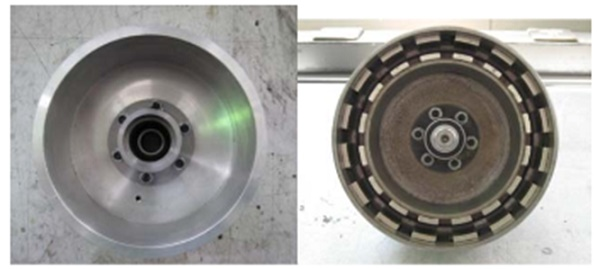Figure 1 - Prototype of the DSPM eddy current coupler [1|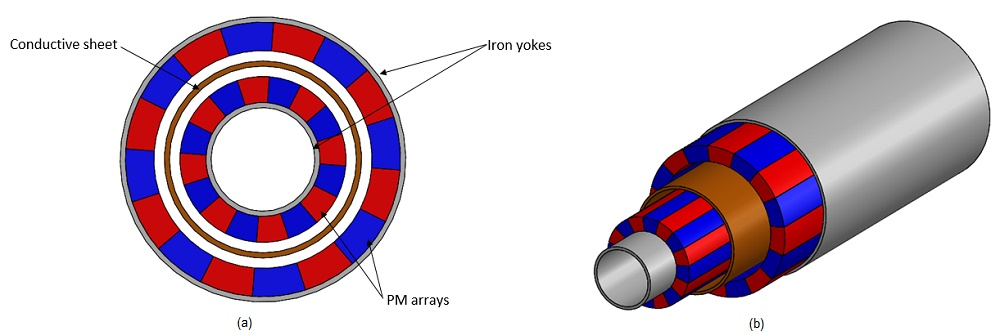Figure 2 - a)- front view and b)- Isometric 3D view of the studied Eddy current coupler.

The materials that are used are summarized in the next table.

Table 1 - Material properties
 Material Magnetic permeability Electrical conductivity (S/m) Remanence Coercivity Iron 4000 10 - PM-NdFeB 0.823 0 1.4 T 1353000 A/m Stainless Steel 304 1 1.37E+6 -

## Results

EMS transient magnetic solver was coupled to SOLIDWORKS Motion and this simulation was used to predict the torque and the eddy losses quantities when an angular speed of 800 rpm is applied to the PM rotors for a simulation time of 75 ms. The transient magnetic simulation with eddy current analysis is shown next.
Figures 3 a) and b) are illustrating the magnetic flux and field density vectors across the studied coupler. Figure 4 shows the Magnetic field intensity at time = 75 milliseconds.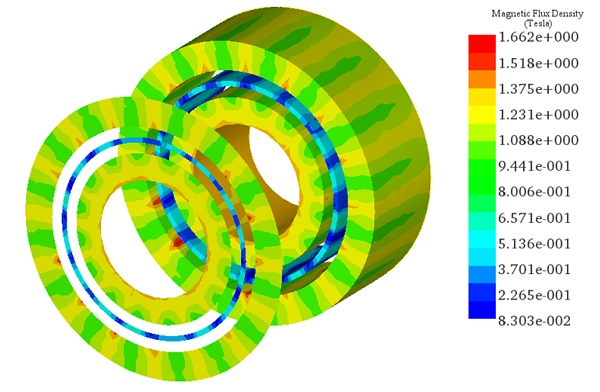(a)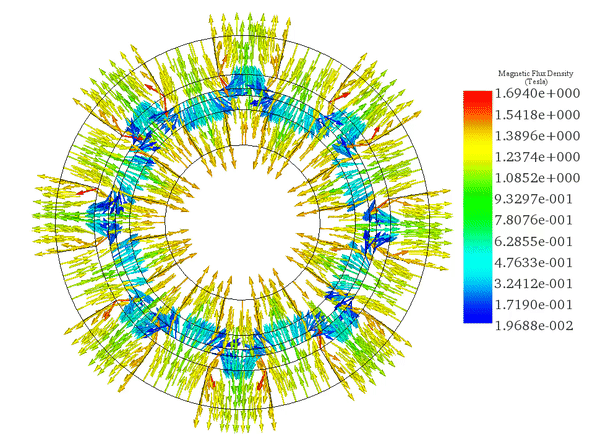(b)
Figure 3 - a)- sectional view of Fringe plot and b)- vector animation of the flux density versus time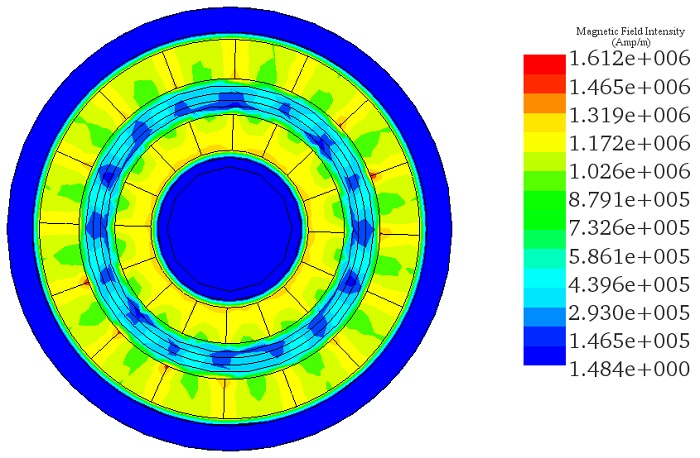Figure 4 - Front Fringe plot of the magnetic field map at t=75ms

Due to the moving magnetic field generated by the rotor, eddy currents are induced inside the conductor part.  The vector plot of those currents across the conductor shows several current loops facing each magnet pole and following the magnetization directions of the PMs.
Since the speed is constant, the eddy currents are remaining relatively invariable during the simulation time as shown in the animation in Figure 5.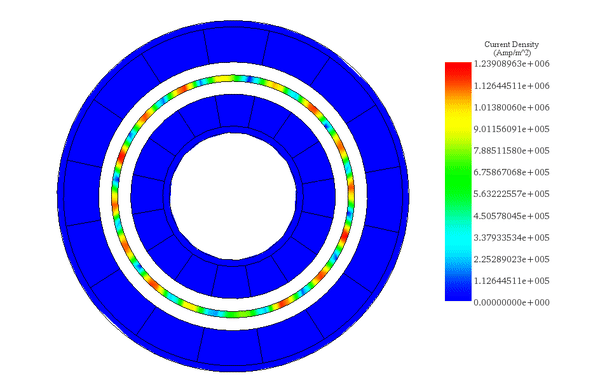(a)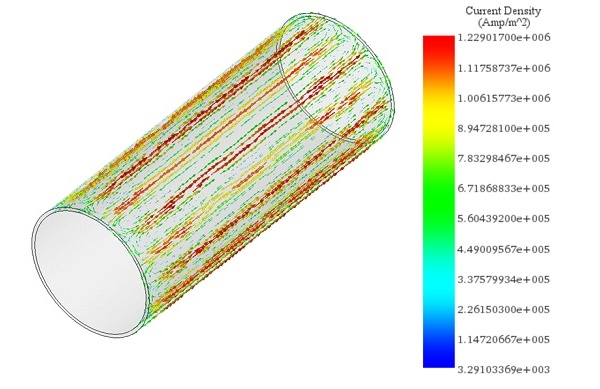Figure 5 - a) GIF animation of eddy current versus time and b) vector plot of surface current loops

The first 2D plot (Figure 6a) shows the constant angular velocity of the PM rotors which has a value of 4800 Deg/s.  Eddy currents generated in the metallic tube will create an electromagnetic torque acting in the reverse direction of the moving PM. The next 2D plot (Figure 6b) shows the induced torque versus time which has an average value around 58.5E-3 N.m.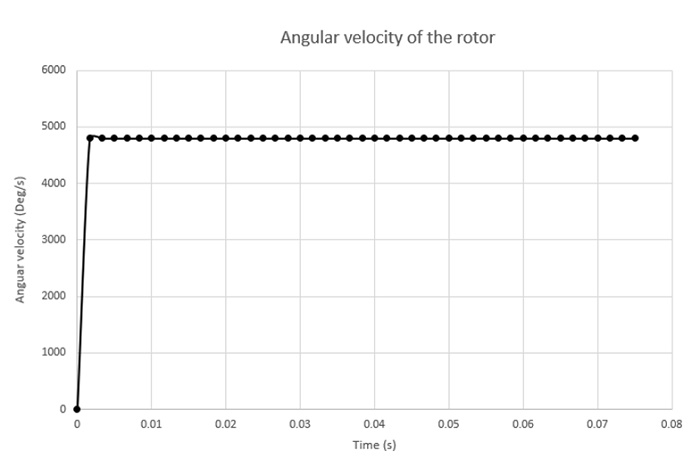(a)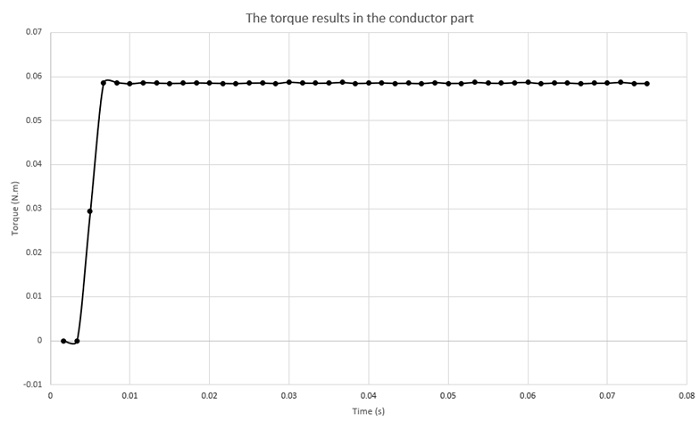(b)
Figure 6 - 2D plot of a) Angular speed of Pm rotors and b)-generated torque across the conduting tube.

Eddy current in a metallic surface mainly has two aspects; a part of eddy current generates inductive magnetic field and another part gets dissipated as heat.  Eddy loss analysis predicted the induced solid losses across the conducting part as shown in the next 2D plot, it has an average value of 5.75 W. Figure 7 shows the solid loss as a function of time. The obtained results show a good agreement with the analytical and experimental results (5.98W and 5.9W respectively) mentioned by the Ref .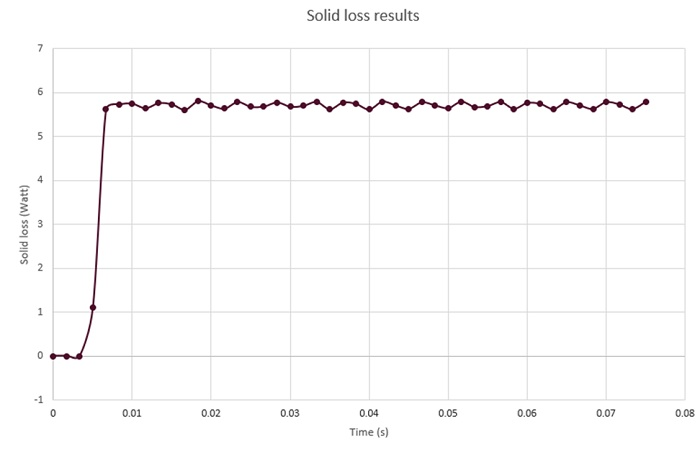Figure 7 - Solid loss results versus time.

Solid losses mainly concentrate in the junction of adjacent eddy current loops which is well displayed by the next fringe plot (Figure 8) of solid loss density across the conductive part at t=75ms.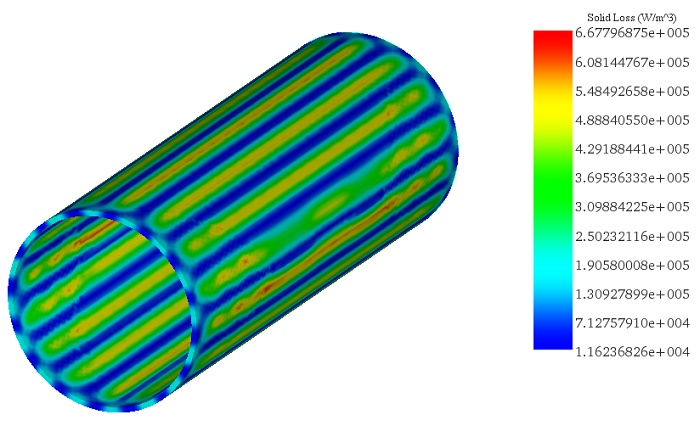Figure 8 - Solid loss density

## Conclusion

Through this study, EMS tool allowed to predict flux density distribution, induced current, eddy losses and generated torque for a radial-flux eddy current Pm coupler under steady state conditions. The obtained results helped to validate the 3D analytical analysis investigated inside the ref . The estimation of induced losses will allow to perform the thermal behavior of such devices in an upcoming works based on EMS tool.

## References

. Erasmus, Abraham Stephanus. Analytical modelling and design optimization of the Eddy Current Slip Coupler. Diss. Stellenbosch: Stellenbosch University, 2017.
 . Zhu, Zina, and Zhuo Meng. "3D analysis of eddy current loss in the permanent magnet coupling." Review of Scientific Instruments 87.7 (2016): 074701.

Upcoming Webinars